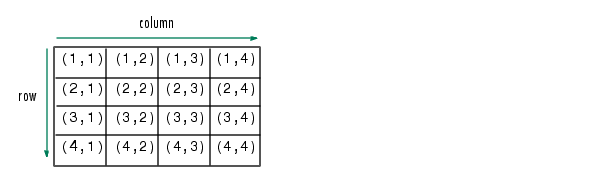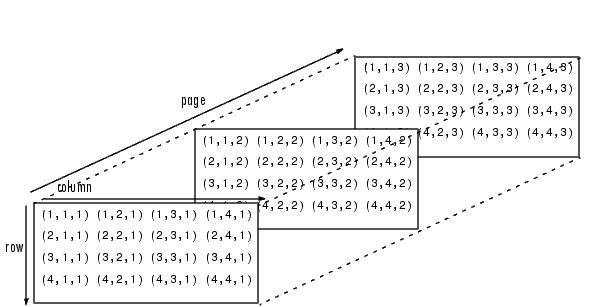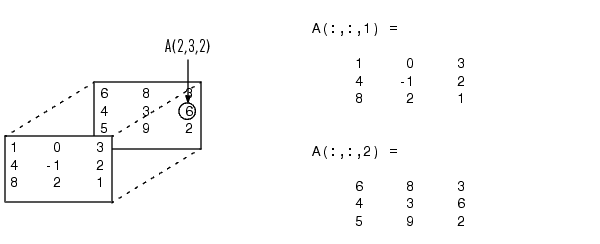ProgrammingMultidimensional Arrays

An array having more than two dimensions is called a multidimensional array in MATLAB. Most of the operations that you can perform on matrices (i.e., two-dimensional arrays) can also be done on multidimensional arrays. This section shows how to create and manipulate these arrays. It covers

Overview

Multidimensional arrays in MATLAB are an extension of the normal two-dimensional matrix. Matrices have two dimensions: the row dimension and the column dimension.You can access a two-dimensional matrix element with two subscripts: the first representing the row index, and the second representing the column index.

Multidimensional arrays use additional subscripts for indexing. A three-dimensional array, for example, uses three subscripts:

• The first references array dimension 1, the row.
• The second references dimension 2, the column.
• The third references dimension 3. This illustration uses the concept of a page to represent dimensions 3 and higher.To access the element in the second row, third column of page 2, for example, you use the subscripts `(2,3,2)`.As you add dimensions to an array, you also add subscripts. A four-dimensional array, for example, has four subscripts. The first two reference a row-column pair; the second two access the third and fourth dimensions of data.

 Note    The general multidimensional array functions reside in the `datatypes` directory.Full and Sparse Matrices Creating Multidimensional Arrays